Subsections

# Numerical Integration

## Purpose

The purpose of this lab is to give you some experience with using the trapezoidal rule and Simpson's rule to approximate integrals.

## Background

In class we have talked about the trapezoidal rule and Simpson's rule for approximating the definite integral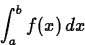Both methods start by dividing the interval [a,b] into n subintervals of equal length by choosing a partition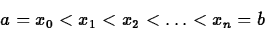satisfying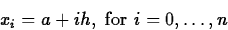whereis the length of each subinterval. For the trapezoidal rule, the integral over each subinterval is approximated by the area of a trapezoid. This gives the following approximation to the integral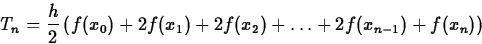There is also an error term associated with the trapezoidal rule that can be used to estimate the error. More precisely, we have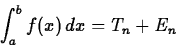where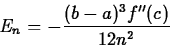for some value c between a and b.

One way to use this error term is as a way to bound the number of subintervals required to achieve a certain tolerance. That is, suppose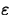is a small number and we want to determine a value of n that guarantees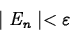If we substitute the error formula from above into this inequality and rearrange it to isolate n2 we get the following.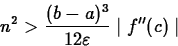Now, if we let M be the maximum of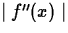on the interval [a,b], we can take the square root of both sides of the equation to obtain the following estimate for n.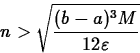The way to think about this result is that it gives a value for n which guarantees that the error of the trapezoidal rule is less than the tolerance. It is generally a very conservative result. As you will discover in the exercises, the actual number of subintervals required to satisfy the tolerance is usually much smaller than the number given by the error estimate.

For Simpson's rule, the function is approximated by a parabola over pairs of subintervals. When the areas under the parabolas are computed and summed up, the result is the following approximation.As for the trapezoidal rule, there is an error formula which says thatwhere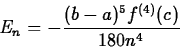for some value c between a and b.

As we did for the trapezoidal rule, we can rearrange this formula to allow us to estimate the number of subintervals required so that we can guaranteeUsing essentially the same steps as we used for the trapezoidal rule, we get the following inequality.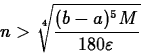where M is the maximum of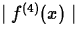on the interval [a,b].

## Exercises

1.
The Getting started worksheet contains two proofs of the following result,which is Simpson's rule applied to the interval [m-h,m+h] using two subintervals. Explain why this result implies the formula given below for Simpson's rule over n subintervals, where n is even.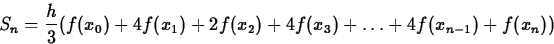2.
For the following functions and intervals, complete the following steps.
(i)
By using Maple's int and, possibly, evalf commands, find a good approximation to the integral of the function over the given interval.
(ii)
Find the minimum number of subintervals required so that Tn satisfies the tolerance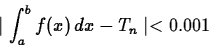Compare this number to the one you would get by using the error estimate for the trapezoidal rule.
(iii)
Find the minimum number of subintervals required so that Sn satisfies the tolerance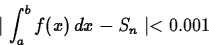Compare this number to the one you would get by using the error estimate for Simpson's rule.
(a)
f(x) = x/(1+x3), interval [0,5].
(b)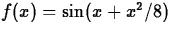, interval [0,10].
3.
Consider the error function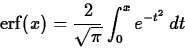Use Simpson's rule to approximateto within an accuracy of 0.1 and determine the minimum number of subintervals required. In a previous lab, you used Taylor polynomials to approximate this same integral. Which method do you think is better? Justify your answer.

4.
Consider the function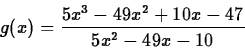Using Simpson's rule, find the minimum number of steps required to approximate each of the two integrals below to within 0.001.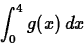andCan you use the error estimate to explain why there is such a big difference between the number of subintervals required?Next: About this document ... Up: No Title Previous: No Title

Jane E Bouchard
2/1/2000